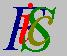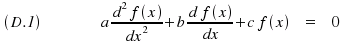Mauro Murzi's pages on Philosophy of Science - Quantum mechanics
Linear differential equation

# [4. Applications.]

## Linear differential equations.

A second order linear differential equation with constant coefficients is an equation of the type:where a , b and c are real numbers.

Finding functions f(x) solutions of this kind of equations is simple. Consider the second order equation a·k² + b·k + c = 0, called the characteristic equation, and let k1 and k2 be its solution. There are three different cases:

1. k1 and k2 are different real numbers. Two particular solutions of equation (D.1) are f1(x) = e x k1 and f2(x) = e x k2 .
2. k1 and k2 are equal real numbers. Two particular solutions of equation (D.1) are are f1(x) = e x k1 and f2(x) = x·e x k1 .
3. k1 and k2 are different complex numbers: k1 = p + iq , k2 = p - iq. Two particular solutions of equation (D.1) are f1(x) = e p x cos(qx) and f2(x) = e p x sin(qx) .

The general solution of equation (D.1) is a linear combination of two particular solutions:  f(x) = A·f1(x) + B·f2(x) , where A and B are real numbers.